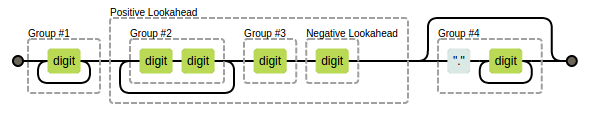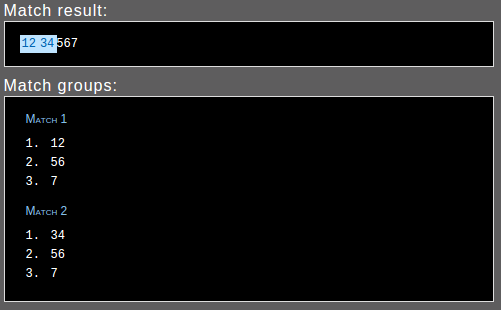• # Revath S Kumar

## Regex: comma seperated indian currency format

04 Nov 2014

Recently, I got a request to show prices in comma seperated format on whatznear.com. Since we are using rails, it have a handy method to do number_to_currency, but unfortunately that was not enough because it follow US system of seperation with thousands. My requirement was to show prices in Indian system of seperation with hundreds.

``````450,500 # US system
4,50,500 # Indian System
``````

So I dropped a mail to Kerala Ruby Users Groups and Hari Sankar replied with a regular expression from his snippet collection.

``````price.to_s.gsub(/(\d+?)(?=(\d\d)+(\d)(?!\d))(\.\d+)?/, "\\1,")
``````

The above regex worked fine for me, but I was curious to know how it works. So I started digging into regex documentation. I am gonna explain, what I understand about the regex.## Group 1 `(\d+?)`

Let look into group 1 ie., `(\d+?)` which says digit should be matched as often as possible. I think there is nothing much confusing here except the tailing `?`. The question mark tells the regex engine, “preceding token zero times or once”. A `?` after `+` makes it lazy and return as soon as the first match.

## positive lookahead `(?=(\d\d)+(\d)(?!\d))`

The Grouping start with `?=` means its a positive lookahead and the previously captured match (matched by group 1) should follow match of this group. Whatever this Group 2 matches won’t expand the match of Group 1.

In order to match this group, 2 digits should be found at least once (`(\d\d)+`), followed by a digit (`(\d)`) and non digit.

`(?!\d)` is a negative lookahead which succeeds when the regex inside lookahead fails. This helps to filter out last 3 digits of a number.

## How Group 1 & positive lookahead works together `(\d+?)(?=(\d\d)+(\d)(?!\d))`

When the group 1 and positive lookahead works together, for the first match there should be a digit followed by at least 1 group of 2 digits, followed by a single digit and non digit.

lets take the number 1234567.00, as the regex engine always returns the leftmost match, the first match will be `12` since it is followed by group of 2 digit (twice) (34 & 56) and a digit then a `.` (non digit). The second match will be `34` since it is followed by group of 2 digit (once) (56) and then a `.` (non digit). Then the engine will try to match again but `56` won’t get a match since it is not followed by the group of 2 digits. So the resulting match will beThe last group `(\.\d+)?` is for floating point.

## String#gsub

Now we have the two matches with first group as `12` and `34` respectively. First group in two matches can be referenced by `\1` since we didn’t name the group. So with String#gsub we can replace the content of group 1 with `<content of group1>,`, ie., we can replace `12` with `12,` and `34` with `34,`.

``````# replacing 12 with 12, and 34 with 34,.
.gsub(/(\d+?)(?=(\d\d)+(\d)(?!\d))(\.\d+)?/, "\\1,")
``````

we used two backslash `"\\1,"` because we used a double quotes, if you are using single quotes just `'\1,'` will be enough. if you didn’t escape the backslash wile using double quotes, ruby will consider `"\1,"` as unicode 1 `\u0001,`.

Hope this explanation helped. Thank you.

If you find my work useful, I appreciate donations via PayPal.